# Surface area of a cube

What is the surface area of a cube that has an edge of 3.5?

S =  73.5

### Step-by-step explanation: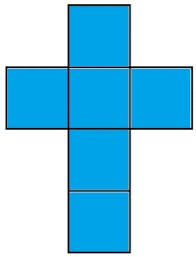Did you find an error or inaccuracy? Feel free to write us. Thank you!## Related math problems and questions:

• Cube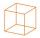Calculate the surface cube with edge 11 dm.
• CubeHow many times increases the surface area of a cube with edge 23.4 cm if the length of the edge doubled?
• Cube 2How many times will increase if the surface area of the cube if we triple length of its edge?
• Special cube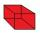Calculate the edge of cube, if its surface and its volume is numerically equal number.
• CubeOne cube has an edge increased five times. How many times will larger it's surface area and volume?
• Cube edgeDetermine the edges of the cube when the surface is equal to 37.5 cm square.
• Cube surface areaThe Wall of the cube has a content area 99 cm square. What is the surface of the cube?
• A butterA butter cube with an edge 6.5 cm long is packed in a package with dimensions a = 28 cm, b = 15 cm. Calculate how many cm2 the package is larger than the surface of the cube.
• Net of a cube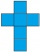We can fold this net to form a cube with a side length of 20 units. What is the surface area of the cube in square units?
• Cube 5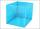The surface of the cube is 15.36 dm2. How will change the surface area of this cube if the length of the edges is reduced by 2 cm?
• The cube - simpleCalculate the surface of the cube measuring 15 centimeters.
• Edge cFind the edge c of cuboid if an edge a = 20 mm, b = 30 mm and surface area S = 8000 mm2.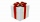How much wrapping paper is needed to wrap a cube-shaped gift with edge measuring 15 cm?The surface of the first cube wall is 64 m2. The second cube area is 40% of the surface of the first cube. Determine the length of the edge of the second cube (x).The volume of the cube is 27 dm cubic. Calculate the surface of the cube.The cube has a surface area of 486 m ^ 2. Calculate its volume.The surface of the cube is 0.54 m2. Calculate the length of the cube edge.